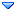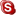GSI Forum
GSI Helmholtzzentrum für Schwerionenforschung

Home » PANDA » PandaRoot » Tracking » RhoError class in pandaroot
RhoError class in pandaroot Tue, 03 June 2014 11:52Elisabetta Prencipe (2) Messages: 214Registered: February 2013 first-grade participant From: *ikp.kfa-juelich.de
Dear Ralf, dear rho-developers,

I have a question how to evaluate the error of the momentum, position, theta, phi, distributions using rho candidates and rho lists, and I am wondering if below is the best way how to proceed. For example, if I want to evaluate the pull of quantities like momentum, or theta and phi, I need information about the reco variables (easy), the true value (easy) and the error distributions. I see that in RhoMath/ the information about the covariance error matrix is accessible. So I could write:

while (theAnalysis->GetEvent() && i++<nevts)
{

theAnalysis->FillList(muminus, "MuonAllMinus");

for (j=0;j<muminus.GetLength();++j)
{

// reco variables
hmomtrk->Fill(muminus[j]->P());
hthttrk->Fill(muminus[j]->P4().Theta());
hphitrk->Fill(muminus[j]->P4().Phi());

// error matrix : variance
RhoError tempvar = muminus[j]->Cov7(); //variance of 7 parameters: x,y,z,px,py,pz,E
double var_px = tempvar(3,3);
double var_py = tempvar(4,4);
double var_pz = tempvar(5,5);
double var_energy = tempvar(6,6);

}
}

This shoud give my access to the variance of px, py, pz, energy. Am I correct?
Sqrt() of what I get from here should deliver the error distribution of px, py, pz, E. Now my question is: is this the way to proceed to get the error distirbutions? and what about Theta() and Phi()? Should I combine, then, the information which I obtain, and get the error distribution for the angular variables? or is there a smarter way/funcion implemented to obtain the error distribution of kinematic variables, in pandaroot?

Elisabetta
Re: RhoError class in pandaroot [message #16767 is a reply to message #16766] Tue, 03 June 2014 11:57Ralf Kliemt Messages: 507Registered: May 2007 Location: GSI, Darmstadt first-grade participantFrom: *gsi.de
Hi Elisabetta,

The RhoCandidate stores the 4-momentum and its covariance matrix in the coordinates of (px, py, pz, e). The covariance is a "RhoError" type, which inherits from TMatrixD. The diagonal elements contain sigma^2 of the corresponding coordinate. If you want to get some error and pull distributions, you can do something like that:

```for (j=0;j<muplus.GetLength();++j)
{
TLorentzVector lv=muplus[j]->P4();
RhoError lvCov=muplus[j]->P4Cov();
hpullpx->Fill( ( lv.Px() - lvmc.Px() ) / sqrt( lvCov ) );
hpullpy->Fill( ( lv.Py() - lvmc.Py() ) / sqrt( lvCov ) );
hpullpz->Fill( ( lv.Pz() - lvmc.Pz() ) / sqrt( lvCov ) );
hpulle->Fill( ( lv.E() - lvmc.E() ) / sqrt( lvCov ) );
}
```

If you need the other coordinates, you would have to convert that error matrix to those coordinates.

Cheers
Ralf
Re: RhoError class in pandaroot [message #16768 is a reply to message #16767] Tue, 03 June 2014 13:12Elisabetta Prencipe (2) Messages: 214Registered: February 2013 first-grade participant From: *ikp.kfa-juelich.de
Hi Ralf,

thank you for your reply. So you confirm that for additional (basic) kimenatic variables nothing is already implemented. Then, I will do myself. no problem!
So, instead of Cov7(), you suggest the use of P4Cov(), where:

cov0] is the variance for px
cov is the variance for py
cov is the variance for pz
cov is the variance for E

Actually I checked, and I found that if I use Cov7(), and I evaluate cov, cov, cov and cov,

or

if I use P4Cov(), as you suggested, to evaluate the variance of px,py,pz,E respectively, as indicated in my previous posting, results are identical, as expected. This is the output for the px variance, if I run in my macro Cov7(3,3) left side, or P4Cov(0,0) right side.

4.7454e-05, 4.7454e-05
7.9324e-05, 7.9324e-05
0.00021817, 0.00021817
0.000140595, 0.000140595
1.7677e-05, 1.7677e-05
0.000121126, 0.000121126
0.000230273, 0.000230273
0.000486443, 0.000486443
2.26079e-05, 2.26079e-05
1.85428e-05, 1.85428e-05
0.000152567, 0.000152567

cheers, Elisabetta
 Previous Topic: genfit in pandaroot - trunk rev 25180: test macros Next Topic: [FIXED] PndTrkTracking2 statusflag array is not initialised
Goto Forum:

Current Time: Thu Oct 05 05:00:26 CEST 2023

Total time taken to generate the page: 0.00879 seconds